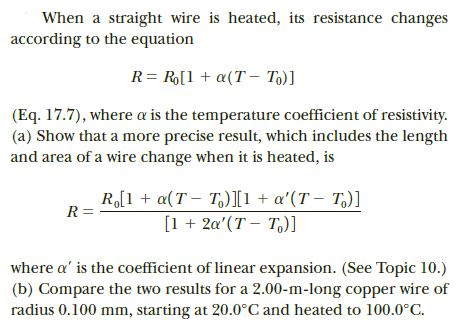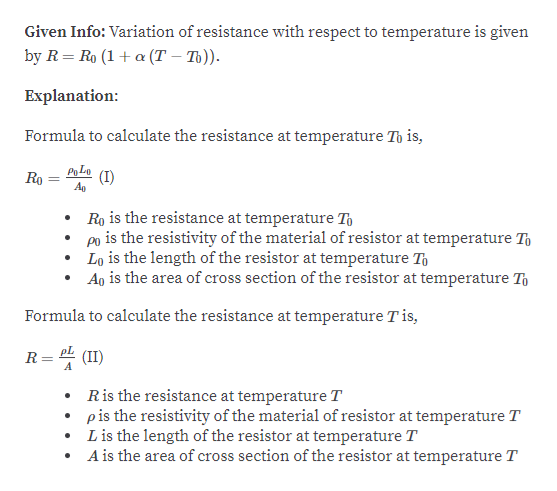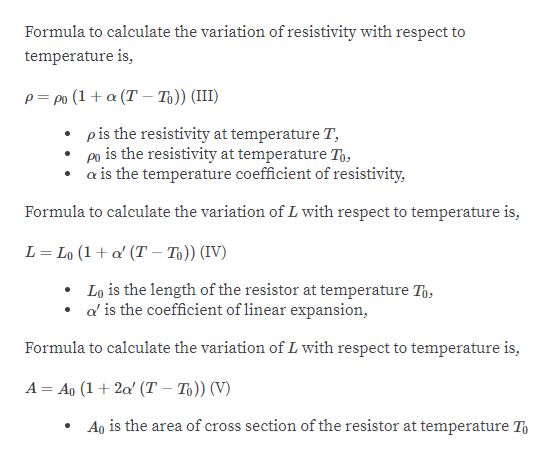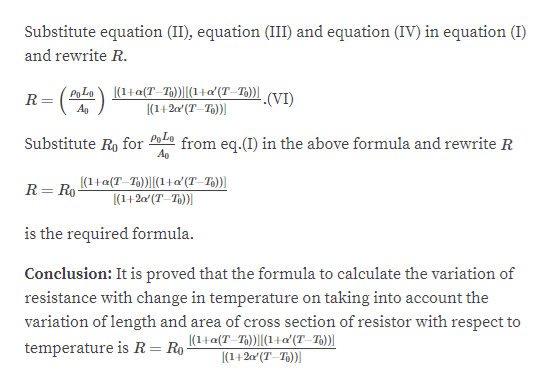# When a straight wire is heated, its resistance changesaccording to the equationR= R[1 + a(T – To)](Eq. 17.7), where a is the temperature coefficient of resistivity.(a) Show that a more precise result, which includes the lengthand area of a wire change when it is heated, isR,[1 + a(T - T)I1 + a'(T - T,)][1 + 2a'(T – T,)]R =where a' is the coefficient of linear expansion. (See Topic 10.)(b) Compare the two results for a 2.00-m-long copper wire ofradius 0.100 mm, starting at 20.0°C and heated to 100.0°C.

Question
1 viewshelp_outlineImage TranscriptioncloseWhen a straight wire is heated, its resistance changes according to the equation R= R[1 + a(T – To)] (Eq. 17.7), where a is the temperature coefficient of resistivity. (a) Show that a more precise result, which includes the length and area of a wire change when it is heated, is R,[1 + a(T - T)I1 + a'(T - T,)] [1 + 2a'(T – T,)] R = where a' is the coefficient of linear expansion. (See Topic 10.) (b) Compare the two results for a 2.00-m-long copper wire of radius 0.100 mm, starting at 20.0°C and heated to 100.0°C. fullscreen
check_circle

Step 1help_outlineImage TranscriptioncloseGiven Info: Variation of resistance with respect to temperature is given by R= Ro (1+ a (T – T)). Explanation: Formula to calculate the resistance at temperature To is, Po Lo Ro (I) Ag Ro is the resistance at temperature To po is the resistivity of the material of resistor at temperature T, Lo is the length of the resistor at temperature To Ao is the area of cross section of the resistor at temperature To Formula to calculate the resistance at temperature T is, pL (II) R Ris the resistance at temperature T pis the resistivity of the material of resistor at temperature T Lis the length of the resistor at temperature T A is the area of cross section of the resistor at temperature T fullscreen
Step 2help_outlineImage TranscriptioncloseFormula to calculate the variation of resistivity with respect to temperature is, p= po (1+ a (T – T6)) (III) pis the resistivity at temperature T, is the resistivity at temperature To, • a is the temperature coefficient of resistivity, po Formula to calculate the variation of L with respect to temperature is, L= Lo (1+ a' (T – Tɔ)) (IV) Lo is the length of the resistor at temperature To, • d'is the coefficient of linear expansion, Formula to calculate the variation of L with respect to temperature is, A= Ao (1+ 2a' (T – To)) (V) Ao is the area of cross section of the resistor at temperature T fullscreen
Step 3help_outlineImage TranscriptioncloseSubstitute equation (II), equation (III) and equation (IV) in equation (I) and rewrite R. PoLo\ [(1+a(T_T.))|(1+a'(T–T3))| |(1+20' (T–T))| .(VI) Ao Po Lo from eq.(I) in the above formula and rewrite R Substitute Ro for |(1+a(T_T3))||(1+a' (T–T3))| (1+2a'(T–Th)| R= Ro is the required formula. Conclusion: It is proved that the formula to calculate the variation of resistance with change in temperature on taking into account the variation of length and area of cross section of resistor with respect to |(1+a(T-T))||(1+a' (T–T))| |(1+2a' (T–T5))| temperature is R=R0 fullscreen

### Want to see the full answer?

See Solution

#### Want to see this answer and more?

Solutions are written by subject experts who are available 24/7. Questions are typically answered within 1 hour.*

See Solution
*Response times may vary by subject and question.
Tagged in

### Current Electricity